• No products in the cart.

# 203.2.1 Logistic Regression, why do we need it?

### Regression Recap

In previous section, we studied about Linear Regression with Multicollinearity in R and Conclusion

• Dependent variable is predicted using independent variables
• A straight line is fit to capture the relation in the form of a model
• The R-Square/ Adjusted R-Square values tell us the goodness of fit of the model
• Once the line is ready we can substitute the values of x(predictor) to get the predicted values of y(dependent variable)

### LAB: Regression – Recap

1. Import Dataset: Product Sales Data/Product_sales.csv
1. What are the variables in the dataset?
1. Build a predictive model for Bought vs Age
1. What is R-Square?
1. If Age is 4 then will that customer buy the product?
1. If Age is 105 then will that customer buy the product?
1. Draw a scatter plot between Age and Buy. Include the regression line on the same chart.

### Solution

1. Import Dataset: Product Sales Data/Product_sales.csv
Product_sales <- read.csv("C:\\Amrita\\Datavedi\\Product Sales Data\\Product_sales.csv")
1. What are the variables in the dataset?
names(Product_sales)
##  "Age"    "Bought"
1. Build a predictive model for Bought vs Age
prod_sales_model<-lm(Bought~Age,data=Product_sales)
summary(prod_sales_model)
##
## Call:
## lm(formula = Bought ~ Age, data = Product_sales)
##
## Residuals:
##      Min       1Q   Median       3Q      Max
## -1.14894 -0.12800 -0.01807  0.10759  1.10759
##
## Coefficients:
##               Estimate Std. Error t value Pr(>|t|)
## (Intercept) -0.1704125  0.0152752  -11.16   <2e-16 ***
## Age          0.0209421  0.0004205   49.80   <2e-16 ***
## ---
## Signif. codes:  0 '***' 0.001 '**' 0.01 '*' 0.05 '.' 0.1 ' ' 1
##
## Residual standard error: 0.1976 on 465 degrees of freedom
## Multiple R-squared:  0.8421, Adjusted R-squared:  0.8418
## F-statistic:  2480 on 1 and 465 DF,  p-value: < 2.2e-16
1. What is R-Square?

0.8421 – 5. If Age is 4 then will that customer buy the product?

new_data<-data.frame(Age=4)
predict(prod_sales_model,new_data)
##           1
## -0.08664394
1. If Age is 105 then will that customer buy the product?
new_data<-data.frame(Age=105)
predict(prod_sales_model,new_data)
##        1
## 2.028511
1. Draw a scatter plot between Age and Buy. Include the regression line on the same chart.
plot(Product_sales$Age,Product_sales$Bought,col = "blue")
abline(prod_sales_model, lwd = 5, col="red")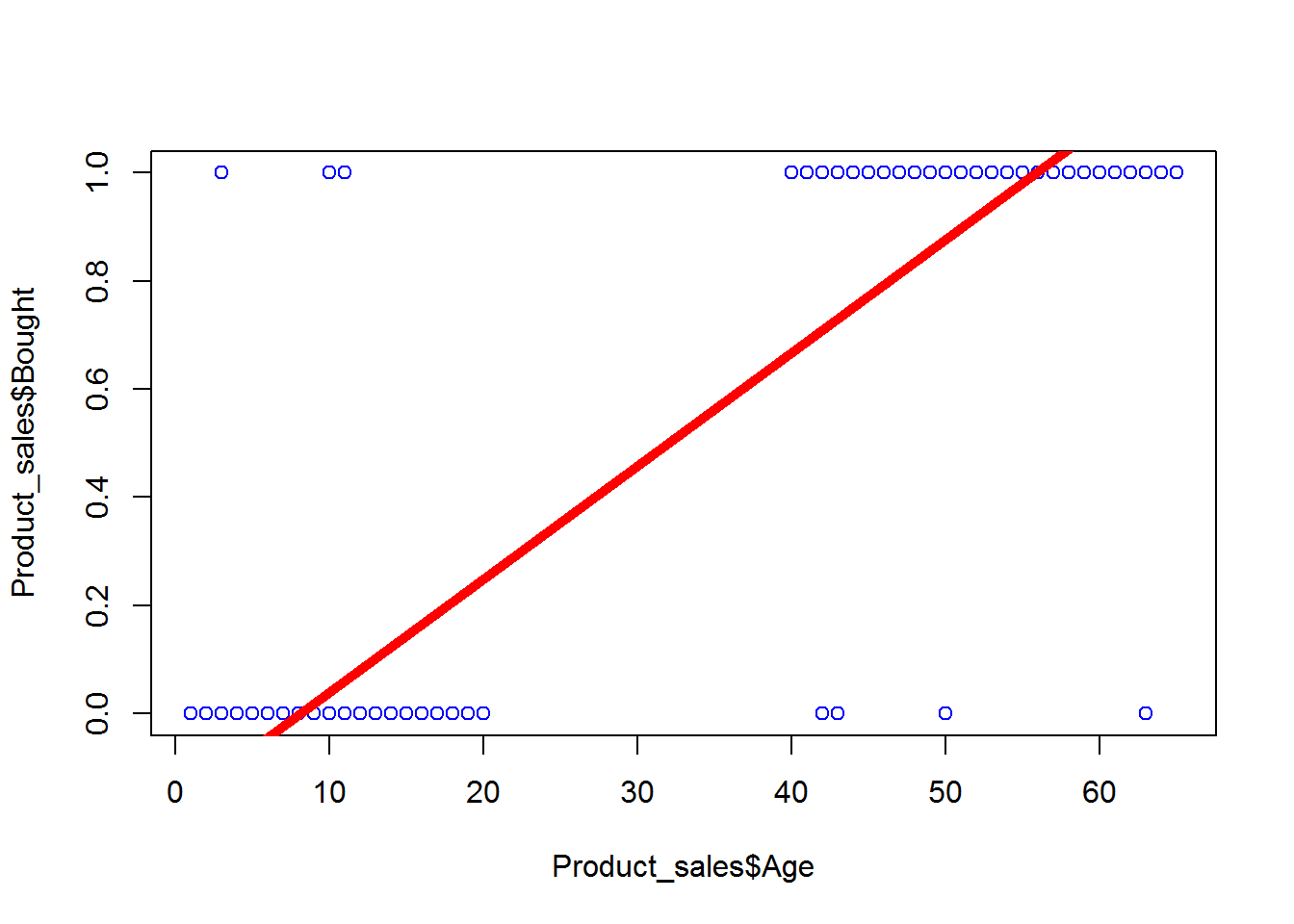### What is the need of logistic regression?

• Consider Product sales data. The dataset has two columns.
• Age – continuous variable between 6-80
plot(Product_sales$Age,Product_sales$Bought,col = "blue")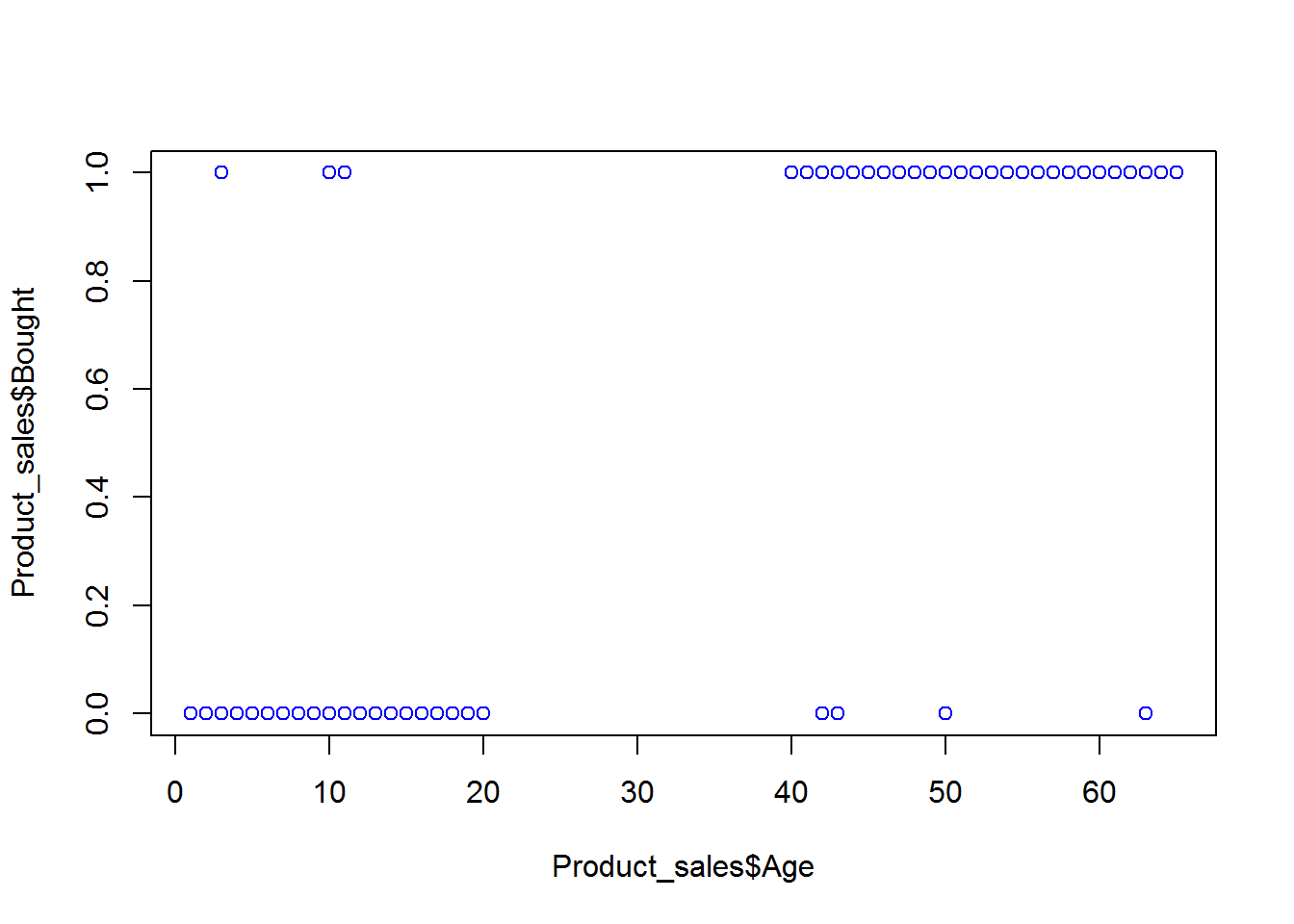#### Real-life examples

• Gaming – Win vs. Loss
• Marketing – Response vs. No Response
• Credit card & Loans – Default vs. Non Default
• Operations – Attrition vs. Retention
• Websites – Click vs. No click
• Fraud identification – Fraud vs. Non Fraud
• Healthcare – Cure vs. No Cure

### Why not linear?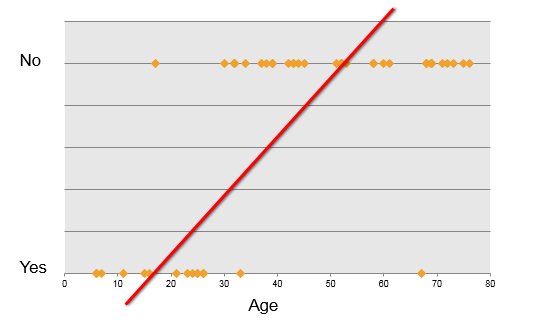### Some Nonlinear Functions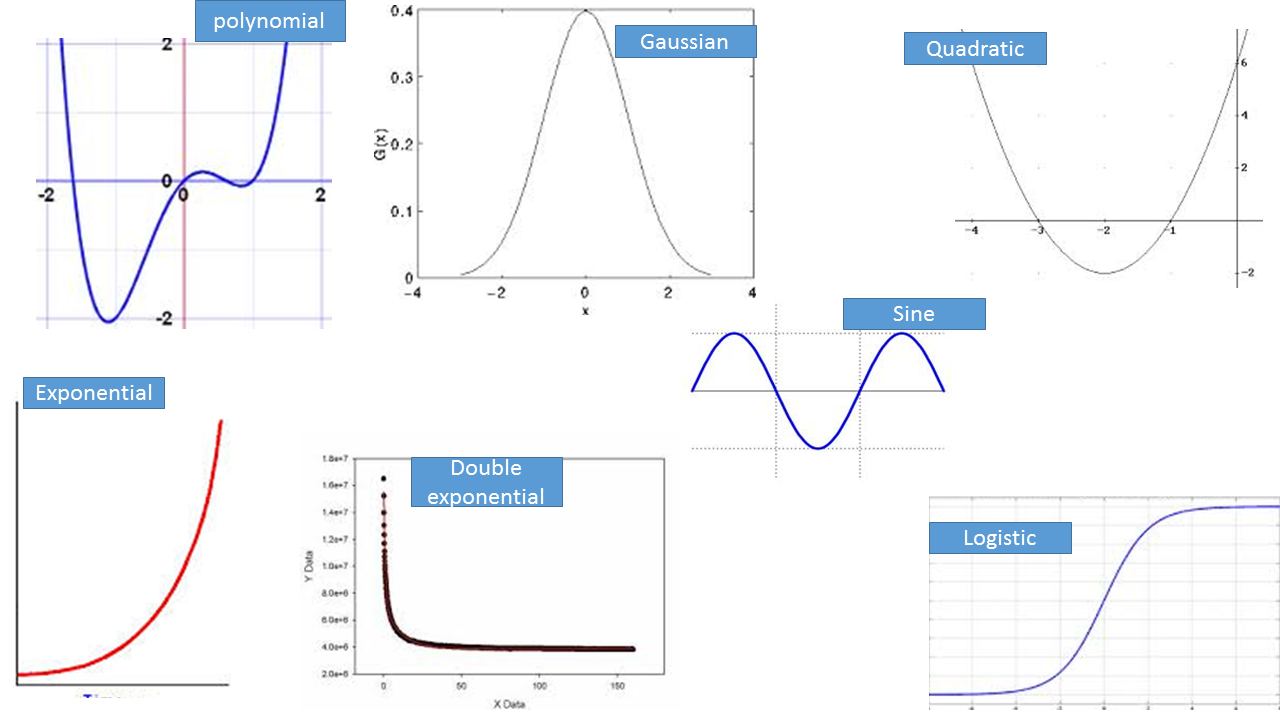### A Logistic Function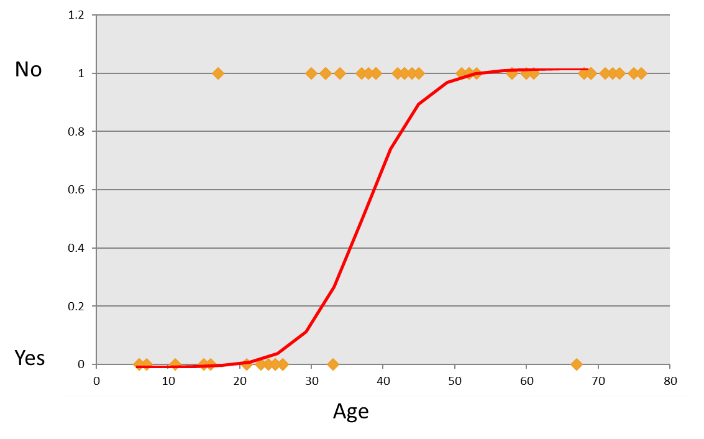### The Logistic Function

• We want a model that predicts probabilities between 0 and 1, that is, S-shaped.
• There are lots of s-shaped curves. We use the logistic model:
• $Probability = \frac{e^{(\beta_0+ \beta_1X)}}{1+e^{(\beta_0+ \beta_1X)}}$
• $log_e(\frac{P}{1-P})=\beta_0+\beta_1X$
• The function on left, $$log_e(\frac{P}{1-P})$$, is called the logistic function.

The next post is about logistic function to regression.

21st June 2017
0 responses on "203.2.1 Logistic Regression, why do we need it?"